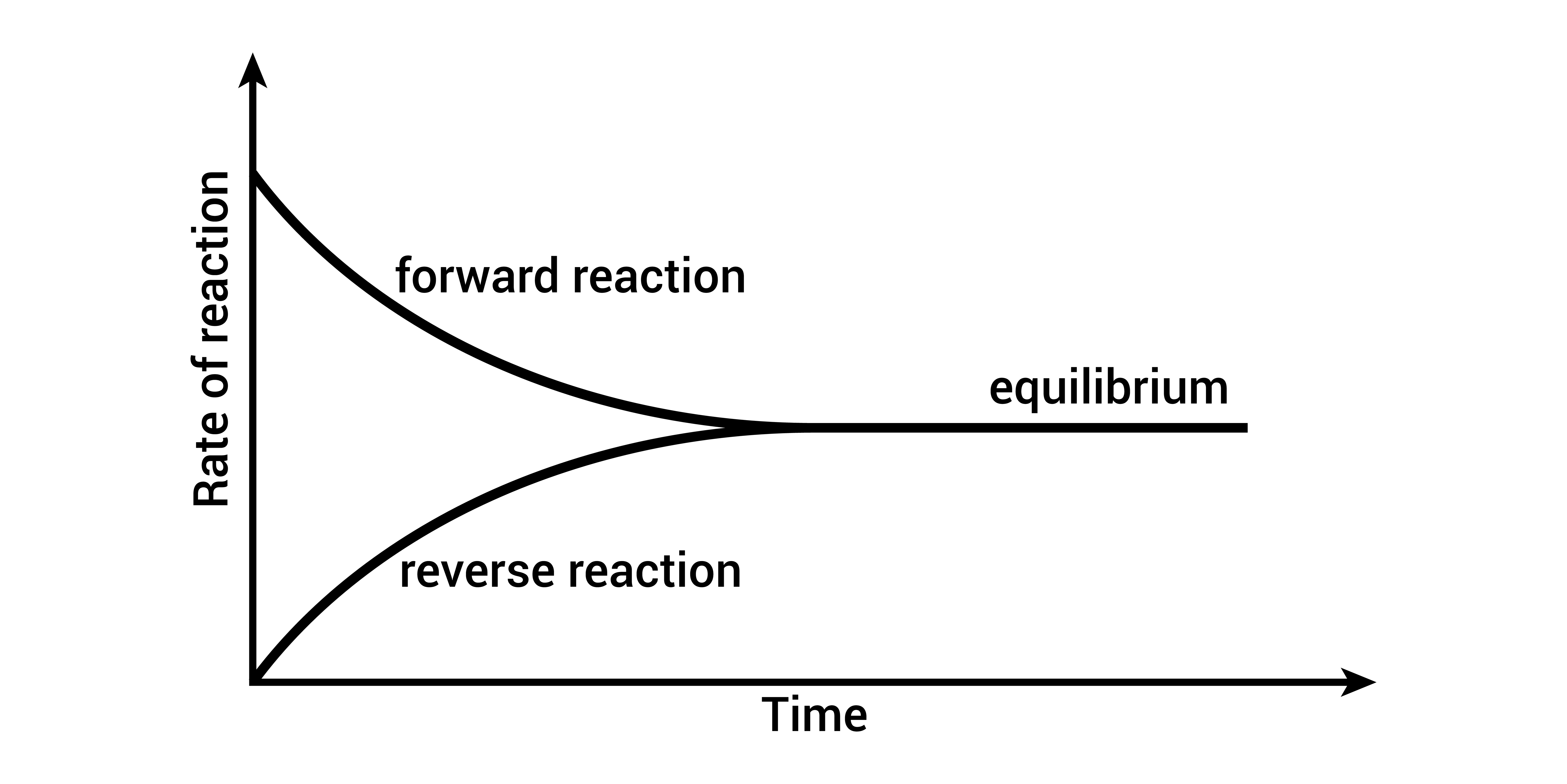×# Introduction

Equilibrium is defined as the state of a system showing that the equilibrium state of a reaction can be affected by changing the temperature, pressure, or concentration of the reactants or products. The state of equilibrium can be influenced by adjusting the system's temperature, pressure, or concentration. When any of these components change, the system's balance is thrown off, and it then readjusts itself until it reaches a new state of stability.

Le Chatelier's principle, commonly known as Chatelier's, is a chemistry principle used to foretell how a shift in environmental factors would affect existing chemical equilibrium constants.

# Types of equilibrium

There are two types of equilibrium in chemistry that are generally studied:

1. Chemical Equilibrium

2. Ionic Equilibrium

In chemistry, ionic and chemical equilibrium play crucial roles. The unionised molecules and ions inside an electrolytic solution are what form ionic equilibrium, whereas the reactants and products of a chemical reaction are what form chemical equilibrium.

# Chemical Equilibrium

In a state of chemical equilibrium, the concentrations of reactants and products are stable over time. It occurs when the forward response rate is identical to the reverse reaction rate. Hence, the reaction is considered to be in equilibrium when the concentrations of the reactants and products are equal.

As a key concept in chemistry, chemical equilibrium describes the states of many different types of chemical reactions. It can also be used to foretell a reaction's outcome and establish the requisite conditions for a reaction.

The rule of mass action is the basis of chemical equilibrium. According to this rule, the reaction rate increases as the product of the reactant concentrations does. Increasing the reactant concentrations leads to a higher reaction rate. On the other hand, the reaction rate drops as reactant concentrations go down.

For a chemical reaction:Here, K is the equilibrium constant.

The equilibrium constant can be used to do more than just predict the result of a reaction; it can also be used to establish the boundary conditions for that reaction.

Increasing the concentrations of the reactants raises the equilibrium constant and shifts the equilibrium towards the products.

On the other hand, if the reactant concentrations are lowered, the equilibrium constant will go down, and the reactants will favor the reaction.Chemical equilibrium graph

# Ionic Equilibrium

The study of ion equilibrium is an important area of study in chemistry. Ion chemistry is a physical chemistry subfield concerned with ions' dynamics and equilibrium in aqueous solutions. The equilibrium between ions in a solution and their behavior can be better understood by first grasping the ionic equilibrium.

When two ions have opposing charges, they are pulled to each other. An ionic connection is formed between two ions in a solution due to their mutual attraction. An ionic equilibrium describes this type of chemical connection.

When unionized molecules and ions in a weak electrolyte solution reach an equilibrium, this state is called ionic equilibrium.

As a rule, the more acidic or basic a solution is, the higher its pH value.

This is due to the presence of hydrogen ions in the solution, which are released by acids.

An ionic equilibrium is established when a sparingly soluble salt is dissolved in water. It is represented asWhen ions are in a state of ionic equilibrium, the quantities of both reactants and products remain constant.

This, however, does not indicate that the reaction has ceased; rather, it indicates that the reaction is continuing in a manner that maintains the status quo of the quantities (the net change is zero).

The fraction of the initial molecules converted at equilibrium is called the degree of Dissociation/ionization. It is calculated asOne other name for ionic equilibrium is "dynamic equilibrium." The reaction is both reversible and continuous in such an equilibrium state. Dynamic equilibrium can only occur in a closed system, in which no energy or matter may leak out.

# Conclusion

Chemical equilibrium is a state in which the concentrations of reactants and products remain constant over time. It is based on the law of mass action, and is used to predict a reaction's outcome and determine the conditions under which a reaction will occur.

1. # When does a chemical equilibrium reaction get affected?

Change in concentration affects chemical equilibrium. When a reaction proceeds in the direction that refills the withdrawn material, the resulting decrease in the concentration of the eliminated reactants or products is alleviated. There is a shift in the chemical equilibrium of a combination if either the reactant or the product concentration is altered.

1. # How does temperature affect equilibrium?

As heat is transferred from one location to another, the temperature rises or falls. Because of this, the chemical equilibria are shifted in favor of the products or reactants, depending on whether the reaction is endothermic or exothermic, respectively.

1. # What do we get if we use Le Chatelier's principle?

Unless the initial circumstances are altered, a stable equilibrium will be maintained in any system. According to Le Châtelier's principle, an equilibrium system will respond to stress in a way that reduces the stress and establishes a new equilibrium.

#### Recent Posts